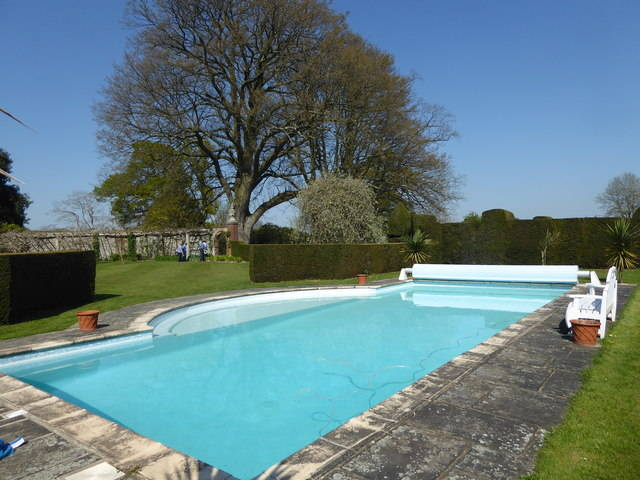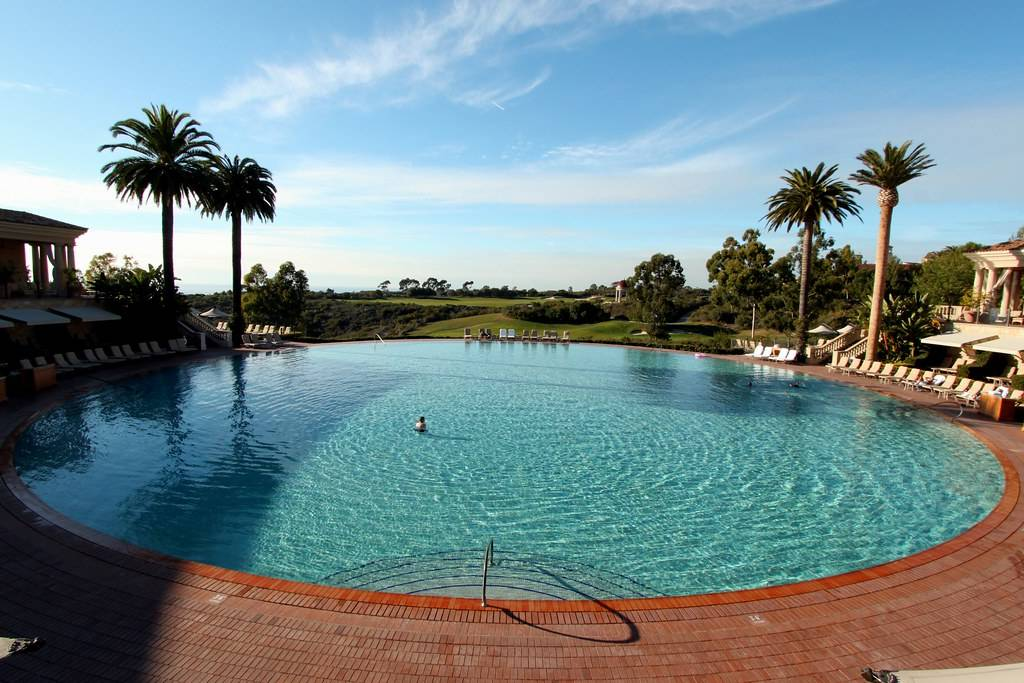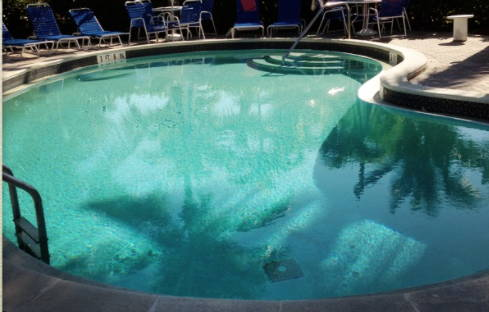First off, we’d like to apologize if this post was a little late in the making when it should have been one of our first posts on the blog. One of the most important things a pool owner should know is the measurement of their pool. The dimensions and the volume. The reason for this is all of your pool calculations, from chemicals, pumps, to filters all depend on the water volume of your pool! But, you know what they say, better late than never!

P.S. We’ve embedded a calculator at the bottom of the page so you don’t have to manually compute the volume of your pool! You’re already going to be doing the hard work of measuring so leave the computations to us!

And remember, measure everything from the inside as we want to get the actual water volume of the pool.

Already have the measurements? Jump Directly to the Calculator!

## How to Compute Volume of a square or rectangular poolYou can get the volume in litres of a square or rectangular pool by multiplying the length by the width and the average depth then multiplying the result by 28.31. To get the average depth of your pool, take the depth of the shallow end of your pool and add it to the depth of the deep end, and divide by two. Here’s a quick example. Let’s say the shallow end of your pool is 3 feet deep and the deep end is 7 feet deep. Add those two together 3 feet +7 feet =10 feet. Divide that by 2, what you’ll end up with is having an average depth of 5 feet.

For Example:

The pool is 30 feet long, 15 feet wide, and has an average depth of 5 feet.

The formula for that would look like:

(30 feet x 15 feet x 5 feet) x 28.31 litres

So that’s 2,250 cubic feet x 28.31 litres

So this particular pool’s volume would be 65,947.5 liters give or take a few.

Length x Width x Average Depth x 28.31 = Volume in litres

For Calculator Use: Take note of these numbers.

Length, Width, Shallow end depth, and Deep end depth

## How to Compute Volume of a round or circular poolTo get the volume, you need to get the radius squared, multiply it by 3.14 then multiply the result by the average depth, then multiply it by 28.31 to get the volume in litres.

Radius squared is basically half of the diameter of your pool, multiplied by itself. So if you have a circular pool that’s 10 feet wide. The diameter will be 10 feet, the radius is half of that, which is 5. Radius squared is 5x5, so this value will be 25.

The formula for this is Radius Squared x 3.14 x average depth x 28.31 = Volume in litres

You can do that, or you can take the diameter and the average depth, and plug it into our handy calculator.

For Calculator use:

Diameter (end to end measurement), average depth

## How to compute volume of an oval pool

First the formula for the volume = length × width × 3.14 ÷ 4 × depth × 28.31

Sound like it’s getting complicated? That’s because it is. The more complex the pool design is, the more factors we have to take into account to get its volume.

From edge to edge, measure the length and the width of your oval pool. Multiply the length and the width by pi (3.14) divide the result by 4, then multiply the result by the average depth. Take the result of that and multiply by 28.31 to get the volume of your pool in litres.

You can do all that, or you can use our calculator, you just need the following information:

Length, width, shallow end depth, and deep end depth.

## Hot to compute volume of a kidney shaped or irregularly shaped poolYou have to take a bunch of measurements when dealing with kidney-shaped or irregularly shaped pools. To get the volume, measure out the length of the pool, measure the short width of the pool (one circular side of the kidney, and the long width of the pool (the later circular side of the kidney) and then multiply the results by .45. Take that result and multiply it by the average depth and then multiply it by 28.31 to get the volume in litres.

Don’t worry if you’re confused, we are too! An easy way to get the volume is to simply measure the length, the short width of the pool, the long width of the pool, the depth on the shallow end and the depth on the deep end. Plug all of the data into the calculator and voila!

## Conclusion

After all those computations, what is the pool volume for? Well, here are a couple of blog posts that can help you put those numbers to good use!

With pool volume in hand, it's now easier to determine dosages in our pool chemistry 101!

You can now determine the correct

See if your pump is undersized and know the perfect pump size you need for your pool.

Use the dimensions to fit for your pool cover!

And so much more!

Thinking of building a pool? Dive into our blog post below for all the must-know details:

Do you have any questions about this topic or the featured products? No worries, we're here to help! Drop us a question down below and we'll get back to you ASAP.

Happy swimming :)

We Need This
We Need This
We Need This